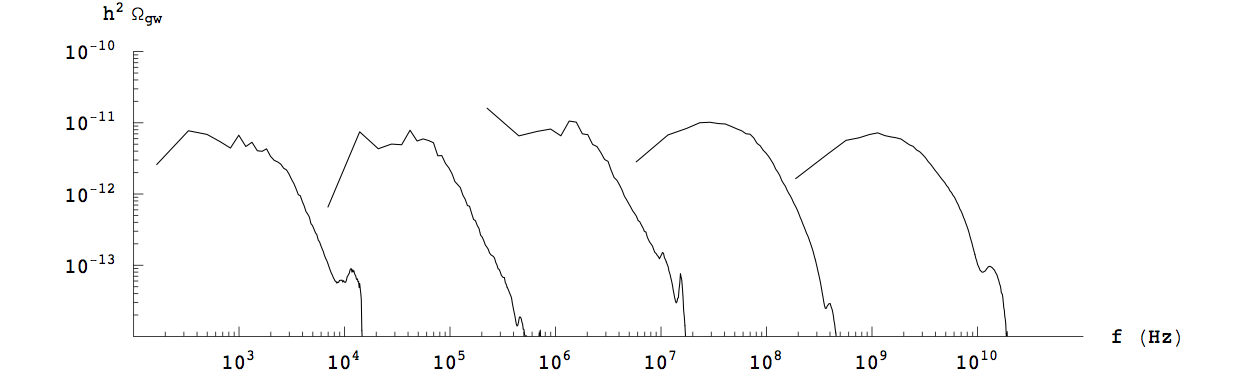## Preheating and Gravitational Waves

I began using numerical simulations to study preheating, an extremely inhomogeneous period that may have existed directly after the end of inflation. It would have occurred as the energy density of the Universe changed from a potential dominated state to a radiation dominated state. In most models, an efficient transition can be obtained through a stage of parametric resonance, during which copious particle production is associated with large gradient energy terms which source gravitational radiation. This radiation immediately decouples from the matter content of the Universe and freely propagates to the present day.

Along with Richard Easther (Yale University) and Eugene Lim (Cambridge University/Kings College London), I designed an algorithm for robustly simulating various early Universe models and predicting the associated gravitational power spectra. From 2006-2008, I wrote and maintained the numerical code and adapted {\sc LatticeEasy} (a publicly available field evolution code) to communicate with our software. This software is one of a few algorithms for the explicit calculation of gravitational wave backgrounds from cosmological scenarios and has become a standard against which newer software is measured.

Many other approaches to calculating cosmological gravitational wave signatures employ Greens' function analysis (Felder:2006cc, GarciaBellido:2007dg, Dufaux:2007pt, GarciaBellido:2007af, Price:2008hq, Dufaux:2008dn) wherein either certain assumptions are made about the expansion of spacetime and exact Greens' Functions can be used or no assumptions are made about the expansion and approximate Greens' Functions are assumed. In both cases the analysis is centered on taking a representative set of wave-vectors and integrating the source term for this finite set. The result is a characteristic power spectrum based on this sampling.

In contrast I have decided to cast the problem as one of more traditional classical field theory (Easther:2006gt,Easther:2007vj). The source term is explicitly known and can be used to source all of the equations of motion of the metric perturbations. Using high-precision computing, these fields can be simulated and the spectrum of gravitational radiation can be explicitly calculated at any time by knowing the full set of the tensor metric perturbations. This calculation is efficient and robust as we employ both configuration space evolution of the non-linear equations of motion that describe the fields as well as spectral methods that evolve the linear equations of motion of the metric perturbations.

The gravitational wave signal from preheating is distinct from the gravitational wave background generated by inflation, which has a nearly scale-invariant power spectrum and an amplitude that depends on the inflationary energy density. The signal from preheating has an approximately fixed amplitude and a peak frequency and shape that depends on the model.

Here we show the effect that changing the energy has on the spectrum of gravitational radiation. This figure assumes that the effective potential at the time of reheating is massive, V(φ) = μ^2φ^2/2, and that the field is coupled to a massless bosonic field, χ, via a four-leg interaction, g^2φ^2χ^2\$.The plot to the right sows the spectrum of gravitational radiation produced during resonance with μ = 10^-18 (left) through to μ = 10^-6 (right) in units where m_Pl ≈ 10^19 GeV = 1, where each spectrum has a value of μ 10^3 times large than the one immediately to the left. The corresponding initial energy densities run from from (4.5x10^9 GeV)^4 to (4.5x10^15 GeV)^4 for our choice of φ_0. The plots are made on 128^3 grids, and the ``feature" at high frequency is a numerical artifact.

We have studied multiple-inflation scenarios (N-flation) in which many fields drive inflation. Thorsten Battefeld (Universit\"at G\"ottingen), Dianna Battefeld (Universit\"at G\"ottingen) and I studied whether resonance can occur in this model, and if so, under what conditions might it occur or be suppressed (Battefeld:2009xw). Later, Xavier Siemens (University of Wisconsin, Milwaukee), Larry Price (California Institute of Technology) and I examined the consequences (Giblin:2010sp) of coupling many fields to the decaying inflationary fields to see if (1) reheating mechanisms are robust to the existence of many types of particles in the early Universe, as should be the realistic scenario and (2) to see if such a signal could give evidence to the number of these fields present at that time.

We also utilized our software to study complex models of multi-field preheating (Battefeld:2012wa). Here we explored what happens when many fields are coupled to a multi-field inflationary model. As the field decays to φ=0 it interacts with many fields whose masses vanish at points in field space that are distributed around (but not at) the origin. We showed that reheating can occur in this model and that energy is distributed among the coupled fields. We further showed that field with ``special" effective masses were more likely to be populated than fields with extremely small masses.